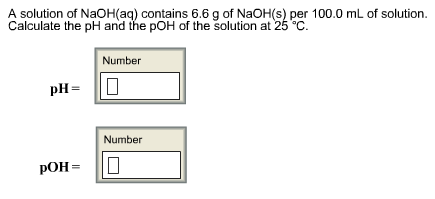# Problem: A solution of NaOH(aq) contains 6.6 g of NaOH(s) per 100.0 mL of solution. Calculate the pH and the pOH of the solution at 25°C. pH = pOH =

🤓 Based on our data, we think this question is relevant for Professor Teets' class at UH.

###### FREE Expert Solution###### Problem Details

A solution of NaOH(aq) contains 6.6 g of NaOH(s) per 100.0 mL of solution. Calculate the pH and the pOH of the solution at 25°C.

pH =

pOH =